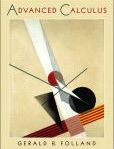Welcome to the IKCEST
Textbook#### Advanced Calculus | Edition: 1

Author: Gerald B. Folland
ISBN: 9780130652652
publisher: Pearson
publisher Date: 12/28/2001
Price: 116.6
eBookPrice: 0
Schools: University at Albany (SUNY)
Description: This book presents a unified view of calculus in which theory and practice reinforces each other. It is about the theory and applications of derivatives (mostly partial), integrals, (mostly multiple or improper), and infinite series (mostly of functions rather than of numbers), at a deeper level than is found in the standard calculus books. Chapter topics cover: Setting the Stage, Differential Calculus, The Implicit Function Theorem and Its Applications, Integral Calculus, Line and Surface Integrals—Vector Analysis, Infinite Series, Functions Defined by Series and Integrals, and Fourier Series. For individuals with a sound knowledge of the mechanics of one-variable calculus and an acquaintance with linear algebra.